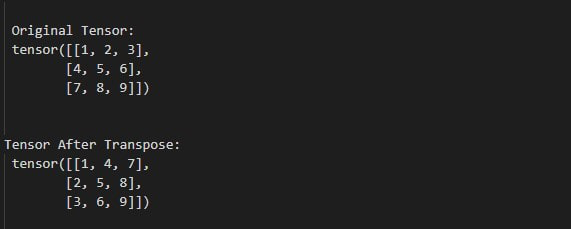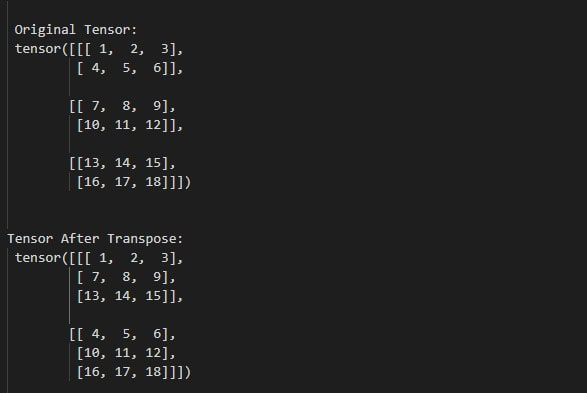Open in App
Not now

# How to find the transpose of a tensor in PyTorch?

• Last Updated : 29 Mar, 2022

In this article, we are going to discuss how to find the transpose of the tensor in PyTorch. The transpose is obtained by changing the rows to columns and columns to rows. we can transpose a tensor by using transpose() method. the below syntax is used to find the transpose of the tensor.

Syntax: torch.transpose(input_tens, dim_0, dim_1)

Parameters:

• input_tens : the input tensor that we want to transpose.
• dim_0 : it will use when we want the first dimension to be transposed..
• dim_1 : it will use when we want the second dimension to be transposed.

Return : this method return transpose of input tensor.

Example 1:

The following program is to understand how to find the transpose of 2D tensor.

## Python

 `# import torch module` `import` `torch`   `# Define a 2D tensor` `tens ``=` `torch.tensor([[``10``, ``20``, ``30``], ` `                     ``[``40``, ``50``, ``60``], ` `                     ``[``70``, ``80``, ``90``]])`   `# display original tensor` `print``(``"\n Original Tensor: \n"``, tens)`   `# find transpose` `tens_transpose ``=` `torch.transpose(tens, ``0``, ``1``)` `print``(``"\n Tensor After Transpose: \n"``, tens_transpose)`

Output:Example 2:

The following program is to know how to find the transpose of multi-dimension tensor.

## Python

 `# import torch module` `import` `torch`   `# Define a 2D tensor` `tens ``=` `torch.tensor([[[``1``, ``2``, ``3``], [``4``, ``5``, ``6``]],` `                     ``[[``7``, ``8``, ``9``], [``10``, ``11``, ``12``]],` `                     ``[[``13``, ``14``, ``15``], [``16``, ``17``, ``18``]]])` `# display original tensor` `print``(``"\n Original Tensor: \n"``, tens)`   `# find transpose of multi-dimension tensor` `tens_transpose ``=` `torch.transpose(tens, ``0``, ``1``)`   `# display final result` `print``(``"\n Tensor After Transpose: \n"``, tens_transpose)`

Output:My Personal Notes arrow_drop_up
Related Articles